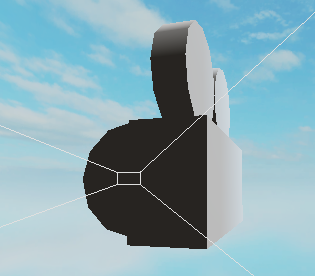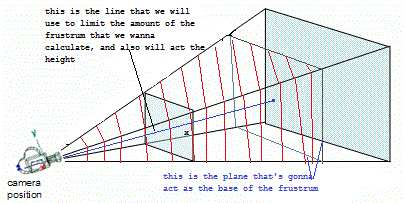# How to find the camera frustum size?

I want to find out how to get the camera frustum.

In other words, how would I find out the size of this little box seen on the rendering test?I would like to know the size for fov 70 but I would also like the equation to figure it out.

1 Like

I’m not sure what you mean. Could you elaborate a bit? Maybe make an illustration showing which “size” you’re trying to figure out.

After I did some searches, a frustrum doesn’t really have a finite size, since it goes on forever even if objects very far away don’t render. So you need to calculate the frustrum’s size from a certain distance of the camera.the equation to calculate the height and width of a frustrum’s base (the plane noted in the picture above) which we will use to calculate the frustum’s size at a given distance, where `distance` is the distance that we chose, (noted as `x` in the picture above)

``````local frustumHeight = 2 * distance * math.tan(math.rad(camera.FieldOfView/2))
``````

Then we can calculate the width simply by doing

``````local frustrumWidth = frustrumHeight / aspect ratio of the camera
``````

And since almost all of the platforms that roblox supports have a `16:9` aspect ration, which is equivilant to `1.78`, we just do

``````local frustrumWidth = frustrumHeight / 1.78
``````

The frustrum’s shape is basically a pyramid with a rectangular base, so in order to calculat its size we need the width and height of the base, then the height of the pyramid.

We have the width and height of the base, now we just need the pyramid’s height, which is simply enough (as noted in the picture above) the distance `x` that we chose.

So, if we just put all those in the equation that’s supposed to calculate a pyramid with a rectangular base’s volume/size. (the size would be a number of course)

``````local size = (frustrumHeight * frustrumWidth * distance)/3
``````

Hope I helped, also I hope this is correct

9 Likes